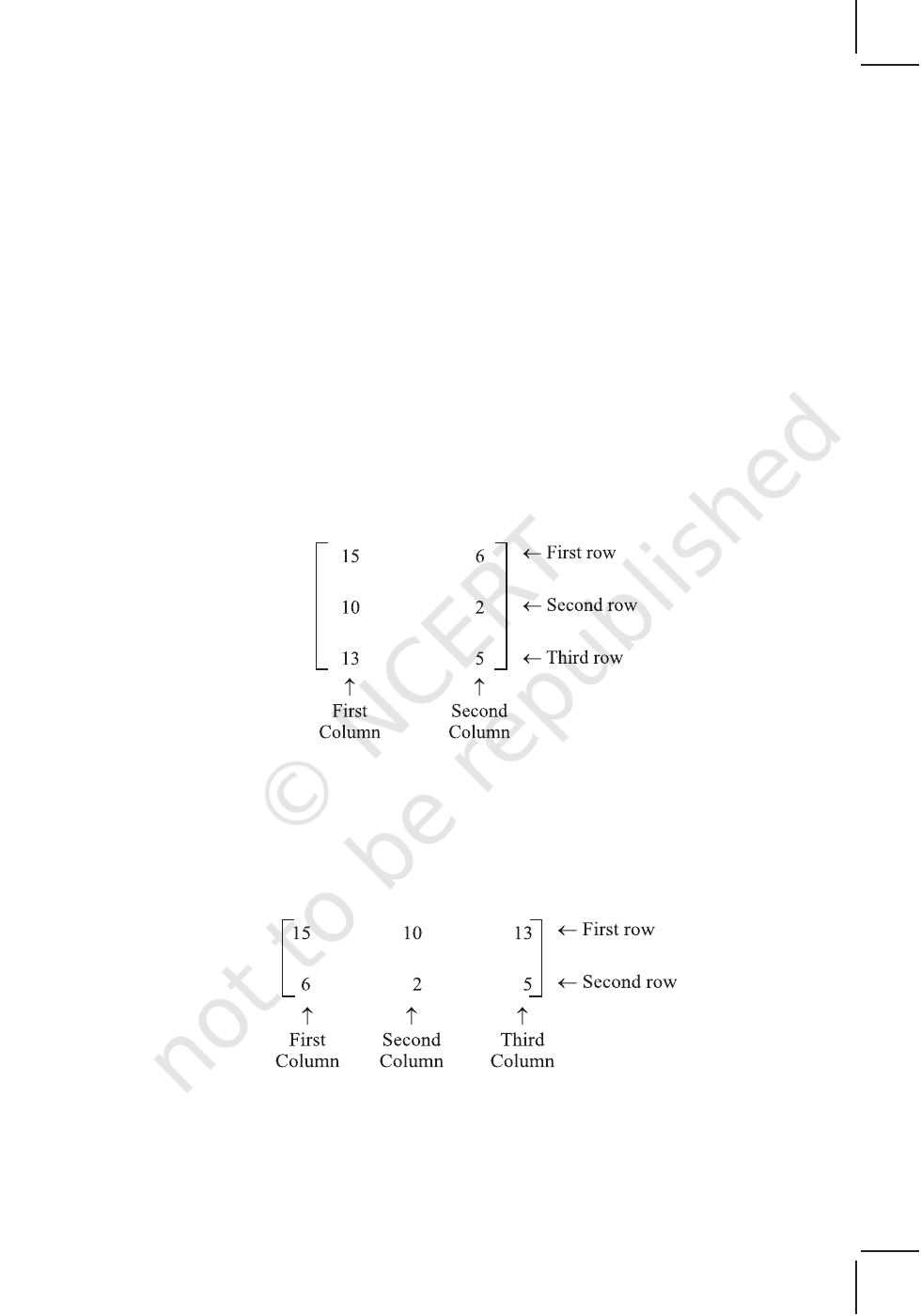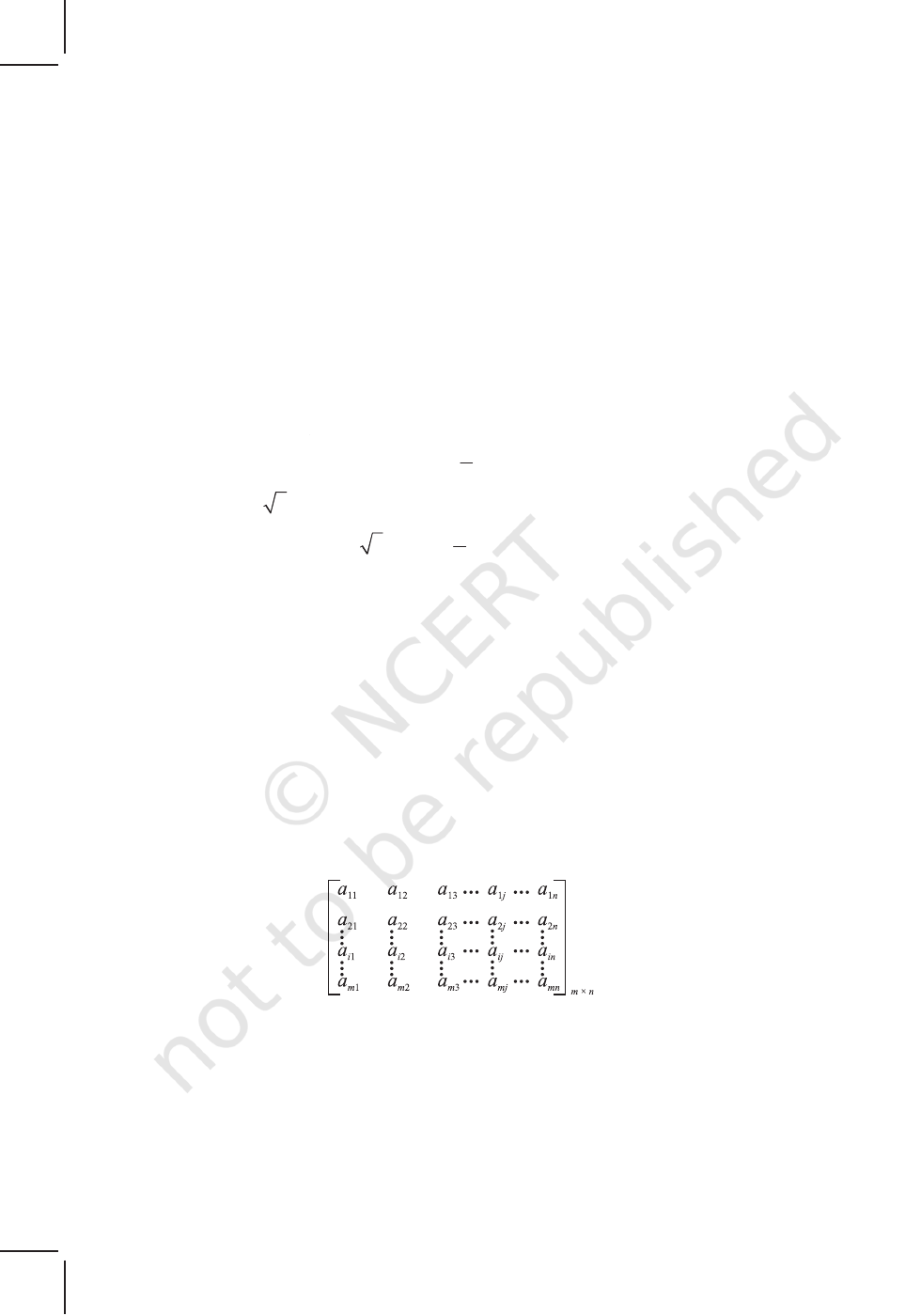56 MATHEMATICS
v
The essence of Mathematics lies in its freedom. — CANTOR
v
3.1 Introduction
The knowledge of matrices is necessary in various branches of mathematics. Matrices
are one of the most powerful tools in mathematics. This mathematical tool simplifies
our work to a great extent when compared with other straight forward methods. The
evolution of concept of matrices is the result of an attempt to obtain compact and
simple methods of solving system of linear equations. Matrices are not only used as a
representation of the coefficients in system of linear equations, but utility of matrices
far exceeds that use. Matrix notation and operations are used in electronic spreadsheet
programs for personal computer, which in turn is used in different areas of business
and science like budgeting, sales projection, cost estimation, analysing the results of an
experiment etc. Also, many physical operations such as magnification, rotation and
reflection through a plane can be represented mathematically by matrices. Matrices
are also used in cryptography. This mathematical tool is not only used in certain branches
of sciences, but also in genetics, economics, sociology, modern psychology and industrial
management.
In this chapter, we shall find it interesting to become acquainted with the
fundamentals of matrix and matrix algebra.
3.2 Matrix
Suppose we wish to express the information that Radha has 15 notebooks. We may
express it as  with the understanding that the number inside [ ] is the number of
notebooks that Radha has. Now, if we have to express that Radha has 15 notebooks
and 6 pens. We may express it as [15 6] with the understanding that first number
inside [ ] is the number of notebooks while the other one is the number of pens possessed
by Radha. Let us now suppose that we wish to express the information of possession
Chapter
3
MATRICES
2019-20MATRICES 57
of notebooks and pens by Radha and her two friends Fauzia and Simran which
is as follows:
Radha has 15 notebooks and 6 pens,
Fauzia has 10 notebooks and 2 pens,
Simran has 13 notebooks and 5 pens.
Now this could be arranged in the tabular form as follows:
Notebooks Pens
Fauzia 10 2
Simran 13 5
and this can be expressed as
or
Notebooks 15 10 13
Pens 6 2 5
which can be expressed as:
In the first arrangement the entries in the first column represent the number of
note books possessed by Radha, Fauzia and Simran, respectively and the entries in the
second column represent the number of pens possessed by Radha, Fauzia and Simran,
2019-2058 MATHEMATICS
respectively. Similarly, in the second arrangement, the entries in the first row represent
the number of notebooks possessed by Radha, Fauzia and Simran, respectively. The
entries in the second row represent the number of pens possessed by Radha, Fauzia
and Simran, respectively. An arrangement or display of the above kind is called a
matrix. Formally, we define matrix as:
Definition 1
A
matrix is an ordered rectangular array of numbers or functions. The
numbers or functions are called the elements or the entries of the matrix.
We denote matrices by capital letters. The following are some examples of matrices:
5
2
A 0 5
3 6
=
,
1
2 3
2
5
3 5
7
i
+
=
,
3
1 3
C
cos tan
sin 2
x
x
x x
x
+
=
+
In the above examples, the horizontal lines of elements are said to constitute, rows
of the matrix and the vertical lines of elements are said to constitute, columns of the
matrix. Thus A has 3 rows and 2 columns, B has 3 rows and 3 columns while C has 2
rows and 3 columns.
3.2.1 Order of a matrix
A matrix having m rows and n columns is called a matrix of order m × n or simply m × n
matrix (read as an m by n matrix). So referring to the above examples of matrices, we
have A as 3 × 2 matrix, B as 3 × 3 matrix and C as 2 × 3 matrix. We observe that A has
3 × 2 = 6 elements, B and C have 9 and 6 elements, respectively.
In general, an m × n matrix has the following rectangular array:
or A = [a
ij
]
m × n
, 1 i m, 1 j n i, j N
Thus the i
th
row consists of the elements a
i1
, a
i2
, a
i3
,..., a
in
, while the j
th
column
consists of the elements a
1j
, a
2j
, a
3j
,..., a
mj
,
In general a
ij
, is an element lying in the i
th
row and j
th
column. We can also call
it as the (i, j)
th
element of A. The number of elements in an m × n matrix will be
equal to mn.
2019-20MATRICES 59
A
Note In this chapter
1. We shall follow the notation, namely A = [a
ij
]
m ×
n
to indicate that A is a matrix
of order m × n.
2. We shall consider only those matrices whose elements are real numbers or
functions taking real values.
We can also represent any point (x, y) in a plane by a matrix (column or row) as
x
y
(or [x, y]). For example point P(0, 1) as a matrix representation may be given as
0
P
1
=
or [0 1].
Observe that in this way we can also express the vertices of a closed rectilinear
figure in the form of a matrix. For example, consider a quadrilateral ABCD with vertices
A (1, 0), B (3, 2), C (1, 3), D (–1, 2).
Now, quadrilateral ABCD in the matrix form, can be represented as
2 4
A B C D
1 3 1 1
X
0 2 3 2
×
=
or
4 2
A 1 0
B 3 2
Y
C 1 3
D 1 2
×
=
Thus, matrices can be used as representation of vertices of geometrical figures in
a plane.
Now, let us consider some examples.
Example 1 Consider the following information regarding the number of men and women
workers in three factories I, II and III
Men workers Women workers
I 30 25
II 25 31
III 27 26
Represent the above information in the form of a 3 × 2 matrix. What does the entry
in the third row and second column represent?
2019-2060 MATHEMATICS
Solution The information is represented in the form of a 3 × 2 matrix as follows:
30 25
A 25 31
27 26
=
The entry in the third row and second column represents the number of women
workers in factory III.
Example 2 If a matrix has 8 elements, what are the possible orders it can have?
Solution We know that if a matrix is of order m × n, it has mn elements. Thus, to find
all possible orders of a matrix with 8 elements, we will find all ordered pairs of natural
numbers, whose product is 8.
Thus, all possible ordered pairs are (1, 8), (8, 1), (4, 2), (2, 4)
Hence, possible orders are 1 × 8, 8 ×1, 4 × 2, 2 × 4
Example 3 Construct a 3 × 2 matrix whose elements are given by
1
| 3 |
2
ij
a i j
=
.
Solution In general a 3 × 2 matrix is given by
11 12
21 22
31 32
A
a a
a a
a a
=
.
Now
1
| 3 |
2
ij
a i j
=
, i = 1, 2, 3 and j = 1, 2.
Therefore
11
1
|1 3 1| 1
2
a
= × =
12
1 5
|1 3 2 |
2 2
a
= × =
21
1 1
| 2 3 1|
2 2
a
= × =
22
1
| 2 3 2 | 2
2
a
= × =
31
1
| 3 3 1| 0
2
a
= × =
32
1 3
| 3 3 2 |
2 2
a
= × =
Hence the required matrix is given by
5
1
2
1
A 2
2
3
0
2
=
.
2019-20MATRICES 61
3.3 Types of Matrices
In this section, we shall discuss different types of matrices.
(i) Column matrix
A matrix is said to be a column matrix if it has only one column.
For example,
0
3
A 1
1/ 2
=
is a column matrix of order 4 × 1.
In general, A = [a
ij
]
m × 1
is a column matrix of order m × 1.
(ii) Row matrix
A matrix is said to be a row matrix if it has only one row.
For example,
1 4
1
B 5 2 3
2
×
=